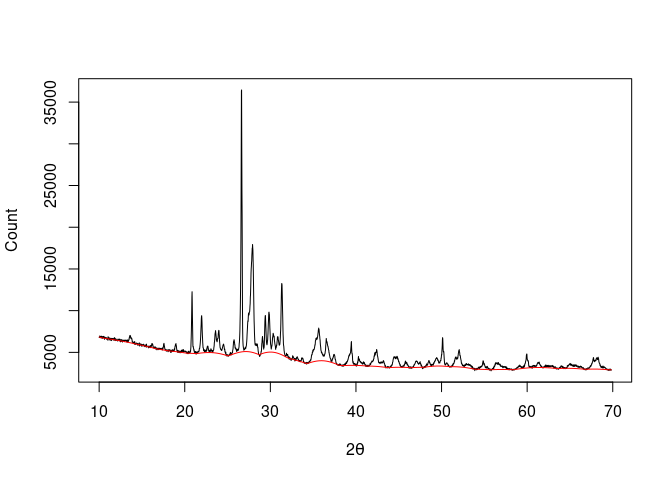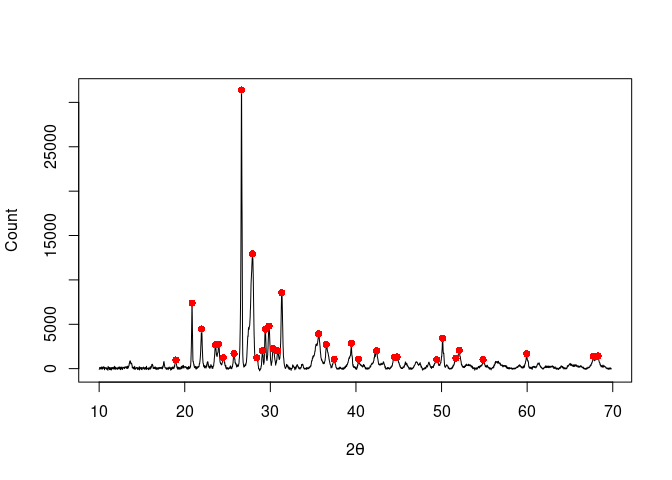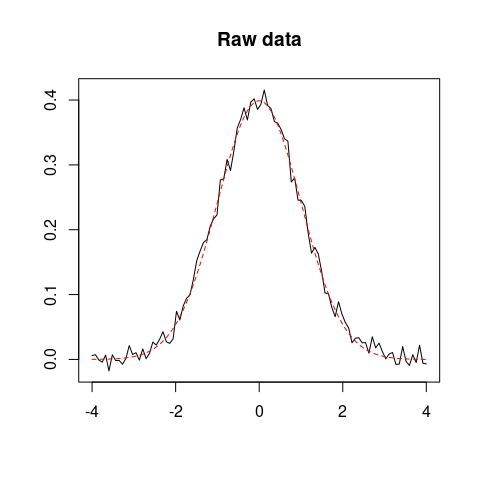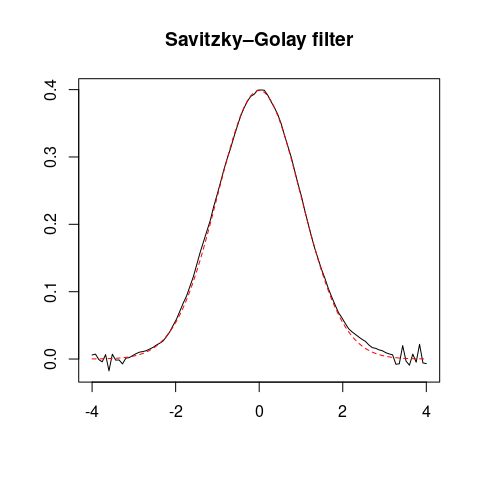## Overview

alkahest is a lightweight, dependency-free toolbox for pre-processing XY data from experimental methods (i.e. any signal that can be measured along a continuous variable). It provides methods for baseline estimation and correction, smoothing, normalization, integration and peaks detection.

## Installation

You can install the released version of alkahest from CRAN with:

install.packages("alkahest")

And the development version from GitHub with:

# install.packages("remotes")
remotes::install_github("tesselle/alkahest")

## Usage

## Load the package
library(alkahest)

alkahest expects the input data to be in the simplest form (a two-column matrix or data frame, a two-element list or two numeric vectors).

## X-ray diffraction
data("XRD")

## Whittaker smoothing
smooth <- smooth_whittaker(XRD, lambda = 1000, d = 2, sparse = TRUE)

## 4S Peak Filling baseline
baseline <- baseline_peakfilling(smooth, n = 10, m = 5, by = 10)

plot(XRD, type = "l", xlab = expression(2*theta), ylab = "Count")
lines(baseline, type = "l", col = "red")## Correct baseline
XRD <- signal_drift(XRD, lag = baseline, subtract = TRUE)

## Find peaks
peaks <- peaks_find(XRD, SNR = 3, m = 11)

plot(XRD, type = "l", xlab = expression(2*theta), ylab = "Count")
lines(peaks, type = "p", pch = 16, col = "red")## Simulate data
set.seed(12345)
x <- seq(-4, 4, length = 100)
y <- dnorm(x)
z <- y + rnorm(100, mean = 0, sd = 0.01) # Add some noise

## Plot raw data
plot(x, z, type = "l", xlab = "", ylab = "", main = "Raw data")
lines(x, y, type = "l", lty = 2, col = "red")

## Savitzky–Golay filter
smooth <- smooth_savitzky(x, z, m = 21, p = 2)
plot(smooth, type = "l", xlab = "", ylab = "", main = "Savitzky–Golay filter")
lines(x, y, type = "l", lty = 2, col = "red")## Contributing

Please note that the alkahest project is released with a Contributor Code of Conduct. By contributing to this project, you agree to abide by its terms.